The following is excerpted from Fluid Flow: A First Course in Fluid Mechanics.  Copyright © 1989 by Macmillan Publishing Company…

4.6 Angular Momentum Theorem

The momentum theorem developed in Section 4.2 gives the force acting on a fixed volume in terms of linear momentum flux through the surface of the volume.  In many situations we are interested in the moment or torque on the volume.  For this purpose we may adapt the angular momentum law of mechanics to the flow of fluids.  Our starting point is the familiar lawwhere m,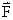, and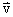refer to a single particle.  The torque exerted by the forceabout a fixed point is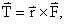where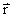is the radius vector from the fixed point to the point of application of.  The symbol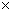signifies, as usual, that the vector cross-product shall be taken.  Then, from Newton’s law of motion,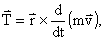We now define a vector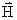as the vector product of the radius vector to the particle and the linear momentum, that is,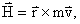(4.8)

The quantityis called angular momentum.  Upon differentiatingwith respect to time, we find that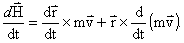However,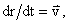and the cross-product of a vector parallel to itself is zero.  The first term in the right-hand side therefore vanishes and we have the result that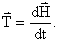(4.9)

Equation (4.9) states that the rate of change of angular momentum of a particle about a fixed point is equal to the torque applied to the particle.

We now seek to modify the law as expressed by Eq. (4.9) to be suitable for a fixed volume.  The development of this equation will therefore follow almost exactly that leading to the momentum theorem of section 4.2.  The torque on a material volume V’ is thenThis is readily transformed into a control volume integral as before, or by the application of Eq. (4.6) upon setting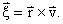We have, therefore,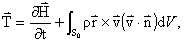(4.10a)

where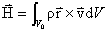(4.10b)

is the angular momentum contained within the control volume.  Equation (4.10a) represents the angular momentum theorem, corresponding to the linear momentum theorem given by Eq. (4.4a).